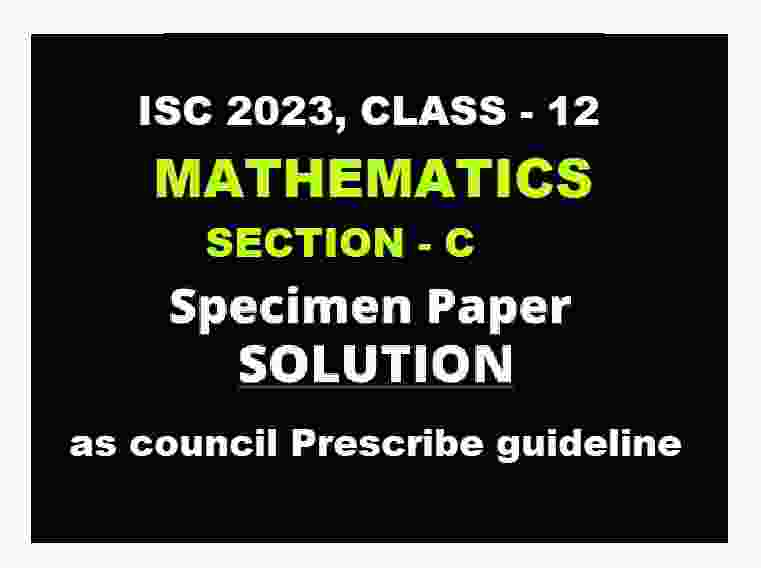Saturday, March 25, 2023

# ISC Maths Specimen Paper 2023 Section C Solved for ISC Class-12

ISC Maths Specimen Paper 2023 Section C Solved for ISC Class-12.  Step by step solutions as council prescribe guideline of model sample question paper.So that student can achieve their goal in next upcoming exam of council. Visit official website CISCE for detail information about ISC Board Class-12..

## ISC Maths Specimen Paper 2023 Section C Solved for ISC Class-12

 Board ISC Class 12th (xii) Subject Maths Topic Specimen Paper Solved Syllabus Revised Syllabus Session 2022-23 Question Type Sec-C Subjective questions Section C (15 Marks) Max mark 80

### ISC Maths Specimen Paper 2023 Sec-C Solved Class-12

Warning :- before viewing solution view Question Paper

### ISC SPECIMEN QUESTION PAPER 2023

MATHEMATICS

Maximum Marks: 80

Time Allowed: Three hours

(Candidates are allowed additional 15 minutes for only reading the paper. They must NOT start writing during this time).

### ISC Maths Specimen Paper 2023 Solved Class-12

Question 19: In subparts (i) and (ii) choose the correct options and in subparts (iii) to (v), answer the questions as instructed.

(a) 𝑅(𝑋) > 𝐶(𝑋)
(b) 𝑅(𝑋) < 𝐶(𝑋)
(c) 𝑅(𝑋) = 𝐶(𝑋)
(d) 𝑅(𝑋) = 2𝐶(𝑋)

#### (ii) If correlation coefficient 𝑟 = 0, then regression lines are

(a) parallel to each other.
(b) not mutually perpendicular.
(c) parallel to coordinate axis.
(d) overlapping lines.

(iii) Average revenue of a commodity is given by 𝐴𝑅(𝑥) = 𝑎 +𝑏/𝑥. Find the demand function when marginal revenue is zero.

(iv) If 𝑅(𝑋) = 36𝑥 + 3𝑥² + 5 then find actual revenue from selling 50th item.

(v) For a given bi-variant distribution, the mean of variable 𝑥 = 4 and the mean of variable 𝑦 = 6. Find the point of intersection of two regression lines.

Question 20: (i) The total revenue function ………. co-ordinate axis.

OR

(ii) For the revenue function 𝑅(𝑥) ……….. g for all 𝑏 < 0 and 𝑎 > 0.

Question 21: The line of regression of marks in Maths (𝑋) and marks in English (𝑌) for a class of 50 students is 3𝑌 − 5𝑋 + 180 = 0. The average score in English is 44 and variance of marks in Maths is 9/16 𝑡ℎ of the variance of marks in English. Find the average score in Maths. Also, find out the coefficient of correlation between marks in Maths and English.

#### Question 22: (i) Solve the following linear programming problem graphically and interpret your result of 𝑍 = 2𝑥 – 5𝑦 subject to the constraints 𝑥 + 𝑦 ≥ 2, 𝑥 − 𝑦 ≥ 0, 𝑥 ≤ 1, 𝑥 ≥ 0, 𝑦 ≥ 0.

OR

(ii) The standard weight of a special purpose brick is 5 kg and it must contain two basic ingredients B1 and B2 . B1 costs ₹ 5 per kg and B2 costs ₹ 8 per kg. Strength considerations dictate that the brick should not contain more than 4 kg of B1 and minimum 2 kg of B2 .Since the demand for the product is likely to be related to the price of the bricks, find the minimum cost of the brick satisfying the above conditions. Formulate this situation as an L.P.P. and solve it graphically.

## ISC Maths Specimen Paper Sec-C PDF Solutions 2023 Class-12

— End of ISC Maths Specimen Paper 2023 Section C Solved for ISC Class-12. :–

–: Visit also :–

Thanks

RELATED ARTICLES